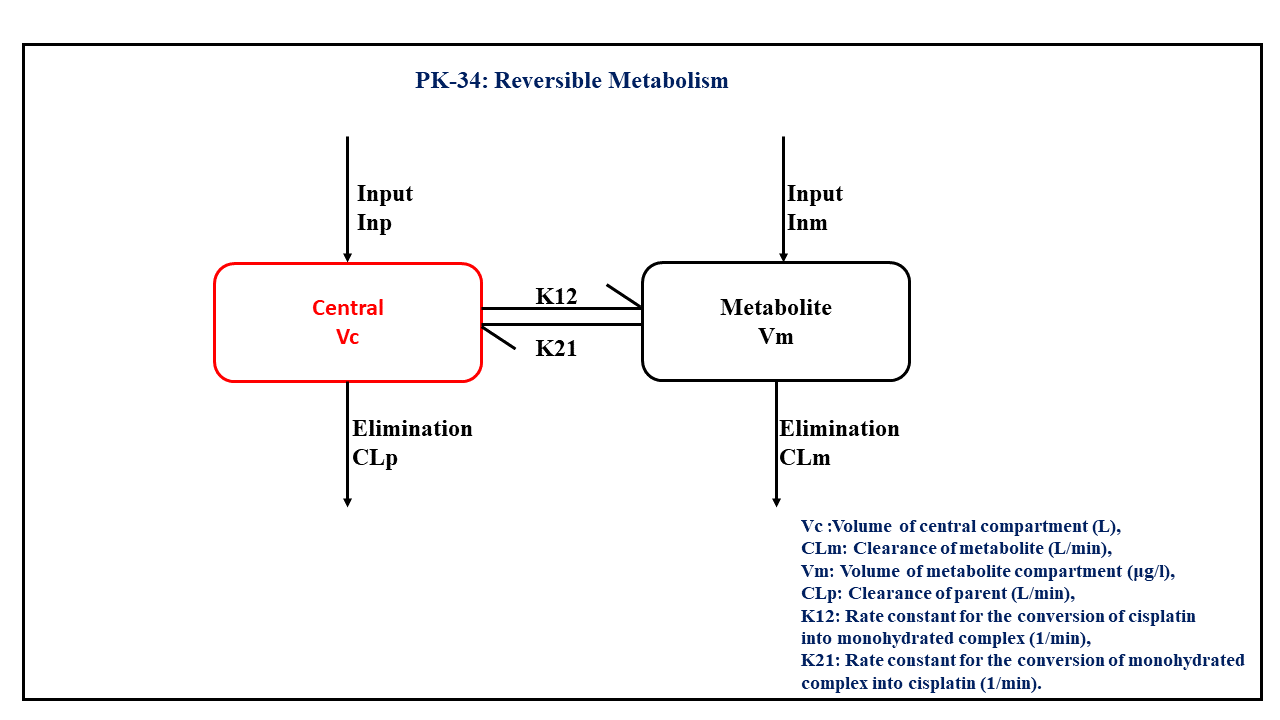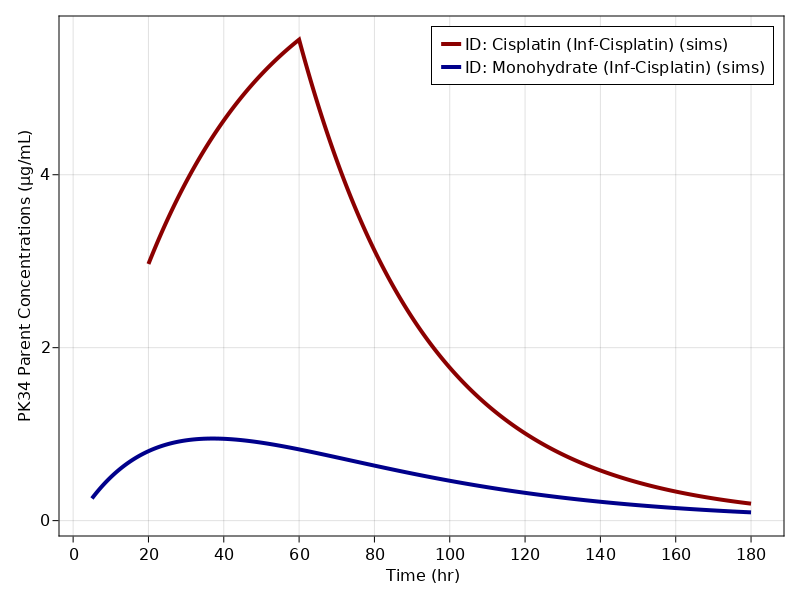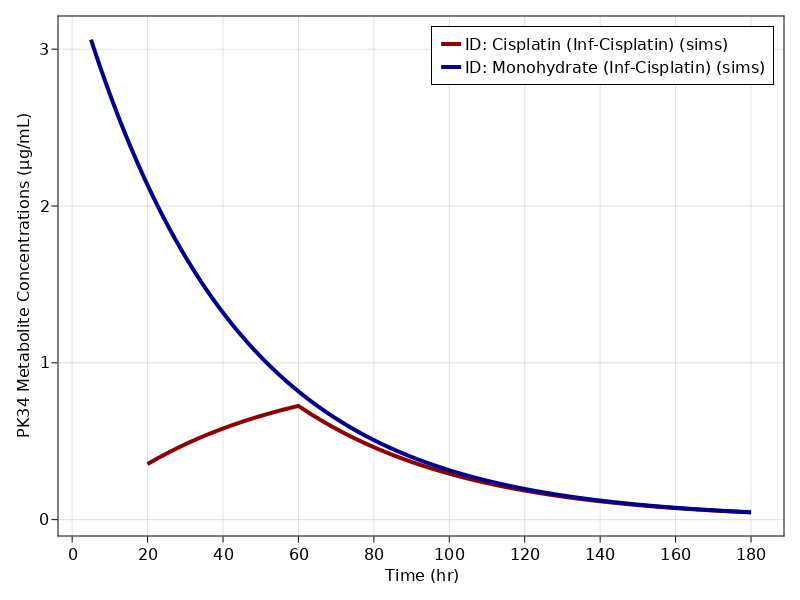# Exercise PK34 - Reversible Metabolism

### Background

• Structural model - Two compartment model

• Route of administration - IV-infusion (with an infusion pump)

• Dosage Regimen - 100 mg/m² of Cisplatin for 1-h at time=0 considering a patient with 1.7m²

• Number of Subjects - 1### Learning Outcome

• We will learn how to simulate kinetics of drug that exhibits reversible metabolism.

• Simulate data for two IV-Infusion with two different rates of infusion regimen.

### Objectives

In this exercise you will learn how to

• Simulate an IV-infusion two compartment model and kinetic of reversible metabolism.

Certain assumptions to be considered:

• A fraction of the dose (2.3%) is present as the monohydrated complex in the infusion solution,

• That there is a reversible reactions between cisplatin (p) and its monohydrated complex (m),

• The input rate can be split into cisplatin infusion rate (Inp) and monohydrate infusion rate (Inm).

### Libraries

Call the "necessary" libraries to get start.

using Random
using Pumas
using PumasUtilities
using CairoMakie


### Model - Microconstant Model

In this two compartment model, we administer the mentioned dose in the Central compartment as well as in the Metabolite compartment at 'time= 0'. Also, $K12$ and $K21$ are the rate constants for the conversion of cisplatin into monohydrated complex and the monohydrated complex into cisplatin, respectively.

pk_34             = @model begin
desc        = "Microconstant Model"
timeu       = u"minute"
end

@param begin
"Volume of Central Compartment (L)"
tvvc        ∈ RealDomain(lower=0)
"Clearance of metabolite (L/min)"
tvclm       ∈ RealDomain(lower=0)
"Volume of Metabolite Compartment (μg/L)"
tvvm        ∈ RealDomain(lower=0)
"Clearance of parent (L/min)"
tvclp       ∈ RealDomain(lower=0)
tvk12       ∈ RealDomain(lower=0)
tvk21       ∈ RealDomain(lower=0)
Ω           ∈ PDiagDomain(6)
"Proportional RUV"
σ²_prop     ∈ RealDomain(lower=0)
end

@random begin
η           ~ MvNormal(Ω)
end

@pre begin
Vc          = tvvc * exp(η)
CLm         = tvclm * exp(η)
Vm          = tvvm * exp(η)
CLp         = tvclp * exp(η)
K12         = tvk12 * exp(η)
K21         = tvk21 * exp(η)
end

@dynamics begin
Central'    = -(CLp/Vc)*Central - K12*Central + K21*Metabolite*Vc/Vm
Metabolite' = -(CLm/Vm)*Metabolite - K21*Metabolite + K12*Central*Vm/Vc
end

@derived begin
cp          = @. Central/Vc
"""
Observed Concentration - Cisplatin (ug/ml)
"""
dv_cp       ~ @. Normal(cp, sqrt(cp^2*σ²_prop))
met         = @. Metabolite/Vm
"""
Observed Concentration - Metabolite (ug/ml)
"""
dv_met      ~ @. Normal(met, sqrt(cp^2*σ²_prop))
end
end

PumasModel
Parameters: tvvc, tvclm, tvvm, tvclp, tvk12, tvk21, Ω, σ²_prop
Random effects: η
Covariates:
Dynamical variables: Central, Metabolite
Derived: cp, dv_cp, met, dv_met
Observed: cp, dv_cp, met, dv_met


### Parameters

Parameters provided for simulation are as below. tv represents the typical value for parameters.

• $Vc$ - Volume of central compartment (L)

• $CLm$ - Clearance of metabolite (L/min)

• $Vm$ - Volume of metabolite compartment (μg/L)

• $CLp$ - Clearance of parent (L/min)

• $K12$ - Rate constant for the conversion of cisplatin into monohydrated complex (min⁻¹)

• $K21$ - Rate constant for the conversion of monohydrated complex into cisplatin (min⁻¹)

• $Ω$ - Between Subject Variability

• $σ$ - Residual error

param = ( tvvc    = 14.1175,
tvclm   = 0.00832616,
tvvm    = 2.96699,
tvclp   = 0.445716,
tvk12   = 0.00021865,
tvk21   = 0.021313,
Ω       = Diagonal([0.0,0.0,0.0,0.0,0.0,0.0]),
σ²_prop = 0.001)

(tvvc = 14.1175, tvclm = 0.00832616, tvvm = 2.96699, tvclp = 0.445716, tvk1
2 = 0.00021865, tvk21 = 0.021313, Ω = [0.0 0.0 … 0.0 0.0; 0.0 0.0 … 0.0 0.0
; … ; 0.0 0.0 … 0.0 0.0; 0.0 0.0 … 0.0 0.0], σ²_prop = 0.001)


### Dosage Regimen

In this section the Dosage regimen is mentioned for:

• Cisplatin Infusion - A total dose of 170mg (100mg/m² * 1.7m²) split as Cisplatin 166.09 and Monohydrate 3.91.

• Monohydrate Infusion - A total dose of 10 mg/L is given as Monohydrate

ev1  = DosageRegimen([166.09,3.91], time = 0, cmt = [1,2], duration = [60,60])
sub1 = Subject(id = "Cisplatin (Inf-Cisplatin)", events = ev1, time = 20:0.1:180)
ev2  = DosageRegimen(10, time = 0, cmt = 2, duration = 2)
sub2 = Subject(id = "Monohydrate (Inf-Cisplatin)", events = ev2, time = 5:0.1:180)
pop2_sub = [sub1, sub2]

Population
Subjects: 2
Observations:


### Simulation

We will simulate the plasma concentration at the pre specified time points.

Random.seed!(123)
sim_sub1 = simobs(pk_34, pop2_sub, param)


### Visualization

f1, a1, p1 = sim_plot(pk_34, sim_sub1,
observations = :cp,
color = :redsblues,
linewidth = 4,
axis = (xlabel = "Time (hr)",
ylabel = "PK34 Parent Concentrations (μg/mL)",
xticks = 0:20:180, ))
axislegend(a1)
f1f2, a2, p2 = sim_plot(pk_34, sim_sub1,
observations = :met,
color = :redsblues,
linewidth = 4,
axis = (xlabel = "Time (hr)",
ylabel = "PK34 Metabolite Concentrations (μg/mL)",
xticks = 0:20:180, ))
axislegend(a2)
f2